# Exponential function facts for kids

Kids Encyclopedia FactsThree different functions: Linear (red), Cubic (blue) and Exponential (green).

In mathematics, an exponential function is a function that quickly grows. More precisely, it is the function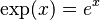$\exp(x) = e^x$, where e is Euler's constant, an irrational number that is approximately 2.71828.

## Properties

Because exponential functions use exponentiation, they follow the same rules. Thus,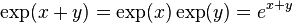$\exp(x+y) = \exp(x)\exp(y) = e^{x+y}$. This follows the rule that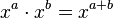$x^a \cdot x^b = x^{a+b}$.

The natural logarithm is the inverse operation of an exponential function.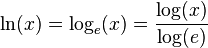$\ln(x) = \log_{e}(x) = \frac{\log(x)}{\log(e)}$

This may also seem unintuitive because of a fundamental property in differential calculus: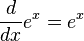$\frac{d}{dx} e^x = e^x$, not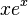$xe^x$ as it would follow the general rule of thumb (see differentiation)

This means that the slope of an exponential function is an exponential function itself, and subsequently this means it has a slope of 1 at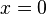$x=0$. These properties are the reason it is an important function in mathematics.

## Applications

The exponential function is among the most useful of mathematical functions. It is used to represent exponential growth, which has uses in virtually all science subjects and it is also prominent in Finance.

## Examples

One example of an exponential function in real life would be interest in a bank. If a person deposits £100 into an account which gets 3% interest a month then the balance each month would be (assuming the money is untouched):

Month Balance Month Balance
January £100.00 July £119.41
February £103.00 August £122.99
March £106.09 September £126.68
April £109.27 October £130.48
May £112.55 November £134.39
June £115.93 December £138.42

Notice how the extra money from interest increases each month. The greater the original balance, the more interest the person will get.

Two mathematical examples of exponential functions are shown below.

## Relation to the mathematical constant e

Even though the base (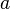$a$) can be any number bigger than zero, for example, 10 or 1/2, often it is a special number called e. The number e cannot be written exactly, but it is almost equal to 2.71828.

The number e is important to every exponential function. For example, a bank pays interest of 0.01 percent every day. One person takes his interest money and puts it in a box. After 10,000 days (about 30 years), he has 2 times as much money as he started with. Another person takes his interest money and puts it back into the bank. Because the bank now pays him interest on his interest, the amount of money is an exponential function. After 10,000 days, he does not have 2 times as much money as he started with, but he has 2.718145 times as much money as he started with. This number is very close to the number e. If the bank pays interest more often, so the amount paid each time is less, then the number will be closer to the number e.

A person can also look at the picture to see why the number e is important for exponential functions. The picture has three different curves. The curve with the black points is an exponential function with a base a little smaller than e. The curve with the short black lines is an exponential function with a base a little bigger than e. The blue curve is an exponential function with a base exactly equal to e. The red line is a tangent to the blue curve. It touches the blue curve at one point without crossing it. A person can see that the red curve crosses the x-axis, the line that goes from left to right, at -1. This is true only for the blue curve. This is the reason that the exponential function with the base e is special.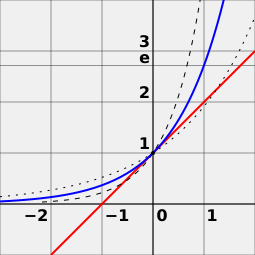e is the unique number a, such that the value of the derivative of the exponential function f (x) = ax (blue curve) at the point x = 0 is exactly 1. For comparison, functions 2x (dotted curve) and 4x (dashed curve) are shown; they are not tangent to the line of slope 1 (red).

## Images for kidsExponential function Facts for Kids. Kiddle Encyclopedia.